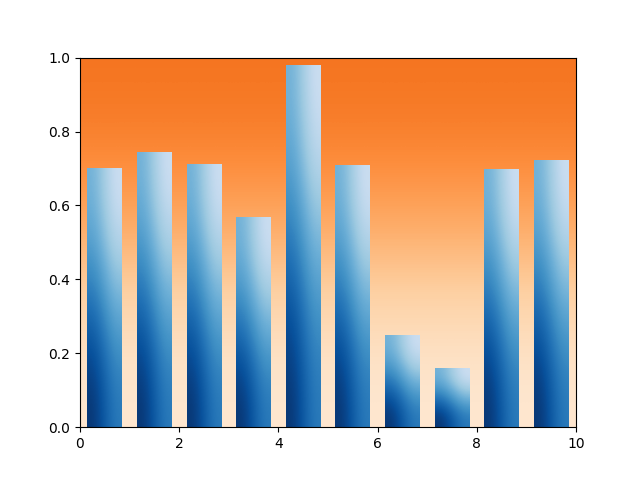# Bar chart with gradients¶

Matplotlib does not natively support gradients. However, we can emulate a gradient-filled rectangle by an AxesImage of the right size and coloring.

In particular, we use a colormap to generate the actual colors. It is then sufficient to define the underlying values on the corners of the image and let bicubic interpolation fill out the area. We define the gradient direction by a unit vector v. The values at the corners are then obtained by the lengths of the projections of the corner vectors on v.

A similar approach can be used to create a gradient background for an axes. In that case, it is helpful to uses Axes coordinates (extent=(0, 1, 0, 1), transform=ax.transAxes) to be independent of the data coordinates.import matplotlib.pyplot as plt
import numpy as np

np.random.seed(19680801)

def gradient_image(ax, extent, direction=0.3, cmap_range=(0, 1), **kwargs):
"""
Draw a gradient image based on a colormap.

Parameters
----------
ax : Axes
The axes to draw on.
extent
The extent of the image as (xmin, xmax, ymin, ymax).
By default, this is in Axes coordinates but may be
changed using the *transform* kwarg.
direction : float
The direction of the gradient. This is a number in
range 0 (=vertical) to 1 (=horizontal).
cmap_range : float, float
The fraction (cmin, cmax) of the colormap that should be
used for the gradient, where the complete colormap is (0, 1).
**kwargs
Other parameters are passed on to .Axes.imshow().
In particular useful is *cmap*.
"""
phi = direction * np.pi / 2
v = np.array([np.cos(phi), np.sin(phi)])
X = np.array([[v @ [1, 0], v @ [1, 1]],
[v @ [0, 0], v @ [0, 1]]])
a, b = cmap_range
X = a + (b - a) / X.max() * X
im = ax.imshow(X, extent=extent, interpolation='bicubic',
vmin=0, vmax=1, **kwargs)
return im

def gradient_bar(ax, x, y, width=0.5, bottom=0):
for left, top in zip(x, y):
right = left + width
gradient_image(ax, extent=(left, right, bottom, top),
cmap=plt.cm.Blues_r, cmap_range=(0, 0.8))

xmin, xmax = xlim = 0, 10
ymin, ymax = ylim = 0, 1

fig, ax = plt.subplots()
ax.set(xlim=xlim, ylim=ylim, autoscale_on=False)

# background image
gradient_image(ax, direction=0, extent=(0, 1, 0, 1), transform=ax.transAxes,
cmap=plt.cm.Oranges, cmap_range=(0.1, 0.6))

N = 10
x = np.arange(N) + 0.15
y = np.random.rand(N)
gradient_bar(ax, x, y, width=0.7)
ax.set_aspect('auto')
plt.show()


Keywords: matplotlib code example, codex, python plot, pyplot Gallery generated by Sphinx-Gallery